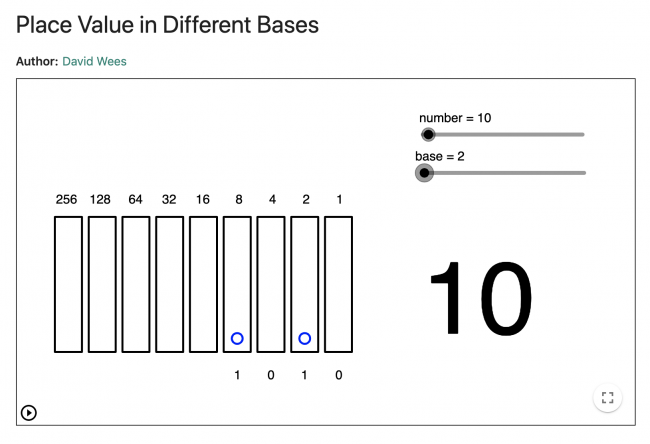# Place Value in Different Bases

Preview:This activity lets students visualize place value in different bases, so as to better understand the base 10 place value relationships of our number system.

Technology
Big Idea:
Grade 4: Development of computational fluency and multiplicative thinking requires analysis of patterns and relations in multiplication and division.
Content:
place value: * 1000s, 100s, 10s, and 1s * understanding the relationship between digit places and their value, to 10 000
Curricular Competency:
Reasoning and analyzing: Use reasoning to explore and make connections
Reasoning and analyzing: Use technology to explore mathematics
Understanding and solving: Develop, demonstrate, and apply mathematical understanding through play, inquiry, and problem solving
Understanding and solving: Visualize to explore mathematical concepts
Communicating and representing: Use mathematical vocabulary and language to contribute to mathematical discussions